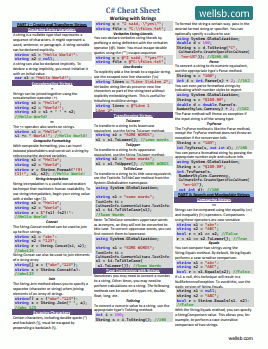# Introduction to Static Variables and Methods

## THE STATIC KEYWORD: BEST PRACTICES AND COMMON MISTAKES

by

In C#, the `static` keyword is used to declare class members that are not tied to a specific instance of the class. Instead, they belong to the class itself. This means that static members can be accessed without creating an instance of the class. In this tutorial, we will discuss static variables and static methods in C#, including code examples, common mistakes, best practices, and more.

## Static Variables in C#

A static variable is a variable that belongs to a class rather than an instance of the class. It is shared among all instances of the class and can be accessed using the class name, rather than an instance of the class.

Here's an example of a static variable in C#:

``````class MyClass
{
public static int staticVar = 0;
}
``````

To access the static variable, you can use the following syntax:

``````int value = MyClass.staticVar;
``````

Static variables can be used for various purposes, such as counting the number of instances created for a class or storing global state information.

### Example: Counting Instances

In this example, we will use a static variable to count the number of instances created for a class:

``````class MyClass
{
public static int instanceCount = 0;

public MyClass()
{
instanceCount++;
}
}

class Program
{
static void Main(string[] args)
{
MyClass obj1 = new MyClass();
MyClass obj2 = new MyClass();
MyClass obj3 = new MyClass();

Console.WriteLine("Total instances created: " + MyClass.instanceCount);
}
}
``````

Output:

``````Total instances created: 3
``````

## Static Methods in C#

A static method is a method that belongs to a class rather than an instance of the class. It can be called using the class name, without creating an instance of the class. Static methods cannot access non-static members of the class, since they do not have access to an instance of the class.

Here's an example of a static method in C#:

``````class MyClass
{
public static void StaticMethod()
{
Console.WriteLine("This is a static method.");
}
}

class Program
{
static void Main(string[] args)
{
MyClass.StaticMethod();
}
}
``````

Output:

``````This is a static method.
``````

## Common Mistakes

1. Trying to access non-static members from a static method: This will result in a compilation error since static methods do not have access to instance-specific data.

``````class MyClass
{
public int nonStaticVar = 5;

public static void StaticMethod()
{
Console.WriteLine("Non-static variable value: " + nonStaticVar); // This will not compile
}
}
``````
2. Accessing static members using an instance: While this is technically allowed in C#, it is considered bad practice and can be confusing.

``````MyClass obj = new MyClass();
int value = obj.staticVar; // Not recommended
``````

## Best Practices

1. Use static members only when necessary: Static members can be useful in certain situations, but overusing them can lead to hard-to-maintain code. Use them only when it makes sense for the member to be shared among all instances of the class.

2. Avoid using static members to store global state: While it might be tempting to use static members to store global state information, doing so can lead to problems such as tight coupling and difficulty in testing. Instead, consider using other patterns, such as dependency injection or a singleton, to manage global state.

3. Do not mix static and non-static members in the same class: Mixing static and non-static members in the same class can lead to confusion and is generally considered bad practice. Instead, separate static and non-static members into different classes, or use a nested static class to group static members together.

``````class MyClass
{
public int nonStaticVar = 5;

public static class StaticMembers
{
public static int staticVar = 0;
public static void StaticMethod() { /* ... */ }
}
}
``````

## The Bottom Line

In this tutorial, you learned about the `static` modifier, specifically its use in declaring static variables and static methods in C#. In addition, you learned how and when to declare static variables and methods, common mistakes, and best practices.

#### Don't stop learning!